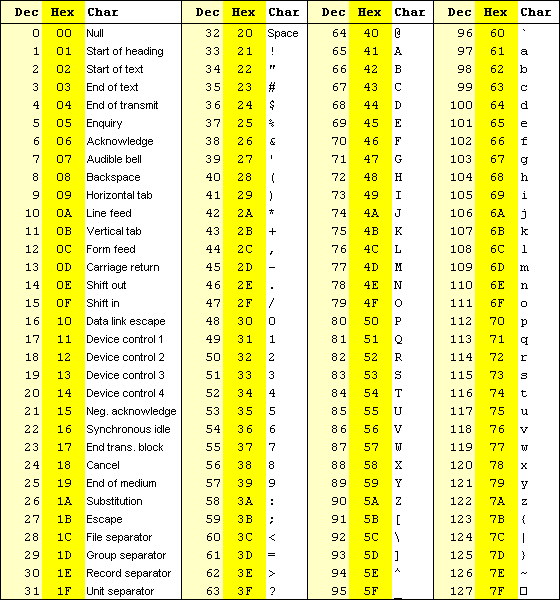ASCII in puzzles:

Martavius had this number string in a puzzle that was the mini-trail to the #jmx (Jaded Media eXperience chat room) TOOC (Taken Out Of Context) page.

11111810111410010111410146104116109

If you're like me you don't know the first thing about ASCII, so you'll have to be told, or guess that the number string represents ascii characters. SpaceBass taught me how to make sense of this kind of puzzle.

First review this two-part ascii chart.Here's the number string again:
11111810111410010111410146104116109

Since there aren't any letters in it, it's safe to guess that the Dec (Decimal) column is the one to try.  The alphabet characters don't begin until number 65. Looking at the numbers in the string, it appears that we're probably dealing with letters rather than strange symbols or numbers.  Since the lowercase letters begin at 97, then try splitting the string into sets of threes.
111 118 101 114 100 101 114 101
The next three number set in the string would be 461.  However the chart only goes to 255.  So now what?  461 isn't valid ascii code.  Try 46 then and see that is code for . (a period or dot) That leaves the final set of three numbers: 104 116 109

111 = o
118 = v
101 = e
114 = r
100 = d
101 = e
114 = r
101 = e
46 = .
104 = h
116 = t
109 = m

overdere.htm
http://www.martavius.com/overdere.htm

It's also possible to use a converter to get this information:
http://www.siteexperts.com/demo/page8.asp

Here's a screen shot of the results when I put 111 into the window and clicked 'Get Character'Using the above chart, I converted overdere.htm to hexadecimal, which looks radically different.
6F766572646572652E68746D
6F = o
76 = v
65 = e
72 = r
64 = d
65 = e
72 = r
65 = e
2E = .
68 = h
74 = t
6D = m

SpaceBass also explained the difference between decimal and hexadecimal to me.

Decimal has 10 digits 0-9 and hex has 16 digits 0-F
Hex is base 16:  0 1 2 3 4 5 6 7 8 9 A B C D E F 10 11 etc.
Because computers are binary logic processors at heart, base 16 is a power of binary. (2x2x2x2)

Let's face it, hexadecimal isn't terribly intuitive for some of us, but I did learn the value of using base 16 in that ten doesn't divide evenly. (10 divided by 2 is 5 but 5 divided by 2 is 2.5).  Still, saying that 6E equals 'n' is just strange to me. It's much easier for me to comprehend lowercase 'n' being the 110th character in the list of code.